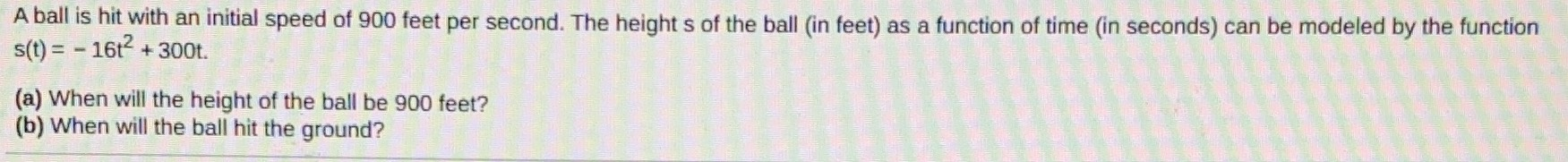### ¿Todavía tienes preguntas de matemáticas?

Pregunte a nuestros tutores expertos
Algebra
PreguntaA ball is hit with an intial speed of $$900$$ feet per second. The height s of the ball (in feet) as a function of time (in seconds) can be modeled by the function $$s ( t ) = - 16 t ^ { 2 } + 300 t$$ .

(a) When will the height of the ball be $$900$$ teet?

(b) When will the ball hit the ground?